# SAT II Math I : Secant, Cosecant, Cotangent

## Example Questions

2 Next →

### Example Question #22 : Trigonometry

Pick the ratio of side lengths that would give sec C.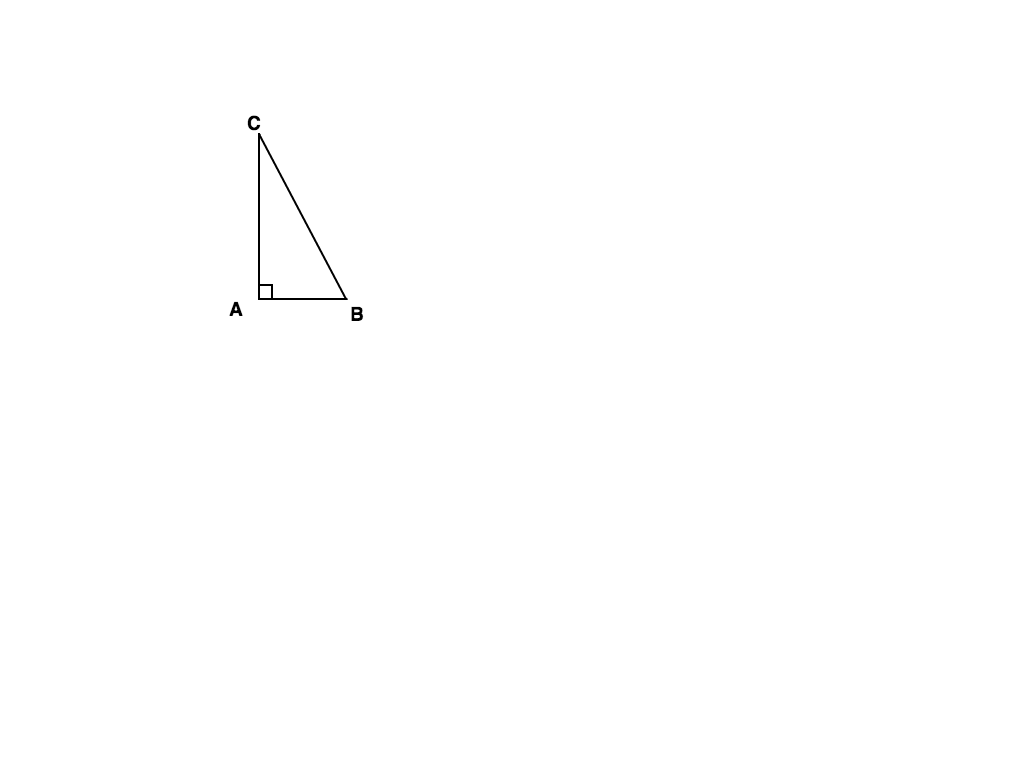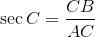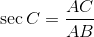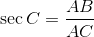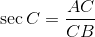Explanation: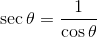Find the ratio of Cosine and take the reciprocal.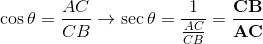### Example Question #23 : Trigonometry

Evaluate: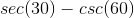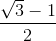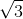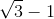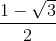Explanation:

Recall that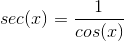and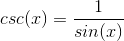.

Rewrite the expression.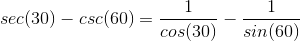The value of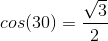and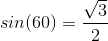.

Since these values are similar, our resulting answer is zero upon substitution.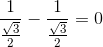The answer is:2 Next →